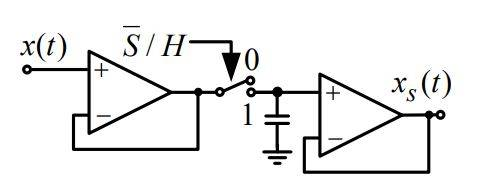# Problem finding the output voltage using Laplace transform

## Homework Statement

The input signal of the circuit shown below is ##x(t)=2\sin (ω_ot + \pi/6)##. The switch in the circuit is controlled with a digital signal of the form ##s(t)=\sum_{k=-\infty}^{+\infty} (u(t+ε-kT_s) - u(t-ε-kT_s))##, ##\frac{2\pi}{T_s}=800\pi##, ##ε\to 0##, so that when the signal equals 1 the switch is in the position 1 (closed).
a) Calculate ##X_s(jω)##
b) If ##g[n]=x_s(nT_s + 0.3T_s)## calculate ##G(jΩ)##.
Note: ##u(t)## is a step funtion, defined as ##u(0)=\frac{1}{2}#### Homework Equations

3. The Attempt at a Solution [/B]
I am not sure how to start and what to do with this digital signal that's controlling the switch. It looks like a sum of delta impulses with very narrow width of ##ε## and a period of ##T_s##. My first thought was that this was some sort of sampling circuit and that i should do a convolution of the two signals to find the ##X_s(jω)##. Something like this:
##x_s(t)=x(t)*s(t)##
##X_s(jω)=\mathscr{L}\{x(t)\} \mathscr{L}\{s(t)\}##. Is this the correct approach?

#### Attachments

Last edited:
•Delta2

marcusl
Gold Member
To start, you need to multiply not convolve. Do you see why? Then you need to consider the action of the second op amp circuit.

•diredragon
rude man
Homework Helper
Gold Member
This ckt is what is called a "boxcar integrator". The input signal is sampled and held until the next sample. So you can forget about the size of ε.

(a) One approach would be to recognize that the output is a series of pulses of width Ts, then recognizing that this output is equivalent to an "ideal" (multiply by δ(t-nT)) sampling of x(t), convolved with a single pulse of width Ts. The latter process is easy since integration with a δ funcion is mathematical pig heaven. Then take the Fourier integral Xs(f) of the output.

(b) let t = nTs - 0.3Ts instead of sin(wt ...) and compute Xs(f) again.

Don't use lapace transform at all.

•diredragon